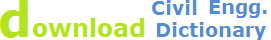compound curves in engineering surveying & levelling, compound horizontal curves

# Compound Curves in Engineering Survey

## Definition:

Combination of two or more simple circular curves of different radius having their curvature in the same direction. Also, A curve that is made up of a series of successive tangent circular arcs is called a compound curve.

Essentially, a compound curve consists of two curves that are joined at a point of tangency and are located on the same side of a common tangent. Though their radii are in the same direction, they are of different values.

tr = perception/reaction time (seconds)f = AASHTO stopping friction coefﬁcient (dimensionless)
e = superelevation,
fs = the coefﬁcient of side friction,
g = the acceleration due to gravity (=9.81 m/s2) and
v = the vehicle speed.
m = distance from centerline to sight obstruction
L = length of curve
S = sight distance (ds)
Rv =Radius to driver’s eye (middle of lane)
T = Tangent Length
C= Length of Chord (Long Chord from PC to PT)
E= External Distance
M = Middle Ordinate
Delta = Deflection Angle

## Elements of a Compound Curve:

The elements of compound curve are:

• PC = point of curvature
• PT = point of tangency
• PI = point of intersection
• PCC  = point of compound curves
• T1 = Length of tangent of the first curve
• T2  = length of tangent of the second curve
• V1 = vertex of the first curve
• V2 = vertex of the second curve
• I1 =  central angle of first curve
• I2 = central angle of second curve
• I angle of intersection = I1 + I2
• Lc1 = length of the first curve
• Lc2 = length of the second curve
• L1 = length of the first chord
• L2 = length of the second chord
• T1 + T2 = length of common tangent measure from V1 to V2
• Θ = 180° – I
• X and Y can be found triangle V1-V2-PI
• L can be found from the triangle PC-PCC-PTSite Map | Civil Engineering Training | Contact Us || © 2014 WebTechTix